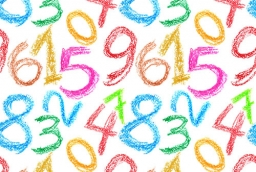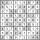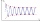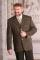# Sum of seven

The sum of seven consecutive odd natural numbers is 119. Determine the smallest of them.

a =  11

### Step-by-step explanation:

a+(a+2)+(a+4)+(a+6)+(a+8)+(a+10)+(a+12)=119

7a = 77

a = 11

Our simple equation calculator calculates it.Did you find an error or inaccuracy? Feel free to write us. Thank you!Tips to related online calculators
Do you have a linear equation or system of equations and looking for its solution? Or do you have a quadratic equation?

## Related math problems and questions:

• Even / odd numbersa / Using variable n write two consecutive odd numbers. b / the sum of three consecutive odd numbers is 333. What are this numbers?
• Odd numbersThe sum of four consecutive odd numbers is 1048. Find those numbers ...
• The sumThe sum of five consecutive odd numbers is 75. Find out the sum of the second and fourth of them.
• Sum of four numbersThe sum of four consecutive natural numbers is 114. Find them.
• SuitThe suit cost 119 euros. How much cost the pants if it is 50% cheaper than the jacket.
• DivisibilityDetermine the smallest integer which divided 11 gives remainder 4 when divided 15 gives remainder 10 and when divided by 19 gives remainder 16.
• Sum of odd numbersFind the sum of all odd integers from 13 to 781.
• Unknown numbersThe sum of two consecutive natural numbers and their triple is 92. Find these numbers.
• AM of three numbersThe number 2010 can be written as the sum of 3 consecutive natural numbers. Determine the arithmetic mean of these numbers.
• Reminder and quotientThere are given numbers A = 135, B = 315. Find the smallest natural number R greater than 1 so that the proportions R:A, R:B are with the remainder 1.
• Wide fieldThe field is 203 meters wide 319 meter long, what is the greater length of the rope by which length and width can be measured and find the exact number of time.
• Divisible by 5How many three-digit odd numbers divisible by 5, which are in place ten's number 3?
• Consecutive numbersSum of ten consecutive numbers is 105. Determine these numbers (write first and last).
• Digit sumDetermine for how many integers greater than 900 and less than 1,001 has digit sum digit of the digit sum number 1.
• Rectangle fieldThe field has a shape of a rectangle having a length of 119 m and a width of 19 m. , How many meters have to shorten its length and increase its width to maintain its area and circumference increased by 24 m?
• Last pageTwo consecutive sheets dropped out of the book. The sum of the numbers on the sides of the dropped sheets is 154. What is the number of the last page of the dropped sheets?
• Digit sumFind the smallest natural number n, the digit sum of which is equal to 37.